# Sale Downpayment Regent Home Bangson 28 Available Foreigner Quota (Owner Post)

Condo
Sale down

฿999,900

(35,711 B./Sq.m.)Updated 7 hoursSee all : 1 Photos🇨🇳 Available Thai / Foreigner Quota ⚠️ Can make an appointment to view the room or request to see the contract ✅ Condo completed in March 2020 ✅ Close to the Purple Line Sky Train, Bang Son Station, only 100 meters away Shut Shuttle Service Shuttle service for children in front of the project ✅ Close to the Si Rat - Outer Ring Road Expressway, just 1.9 km. ☑️ can ask to see the room and contract page Can I ask the house number? ☑️ Price negotiable (N / A) ☑️ Accept Agent 🔘 Contract price 999,900 baht 🔘 Room 28 sq.m., 56 sq.m. and 61 sq.m. ••• Building A ••• 0405 = 1,140,000 0406 = 1,140,000 0412 = 1,140,000 0413 = 1,160,000 0414 = 1,170,000 0440 = 1,200,000 🚩 Wide room 0444 = 1,140,000 0445 = 1,140,000 0446 = 1,160,000 0646 = 1,180,000 0751 = 1,160,000 0905 = 1,180,000 0920 = 1,180,000 0921 = 1,180,000 0929 = 1,180,000 0930 = 1,180,000 0931 = 1,180,000 1046 = 1,220,000 1840 = 1,300,000 🚩 Wide room 1940 = 1,330,000 🚩 Wide room 1945 = 1,250,000 1946 = 1,250,000 2434 = 1,260,000 2444 = 1,250,00 2445 = 1,250,000 2446 = 1,250,000 2511 = 1,300,000 2532 = 1,320,000 1997 = 1,360,000 🚩 Wide room 2003 = 1,330,000 2619 = 1,330,000 2620 = 1,330,000 2621 = 1,330,000 2622 = 1,330,000 2623 = 1,330,000 2624 = 1,330,000 2634 = 1,330,000 2645 = 1,330,000 2740 = 1,360,000 🚩 Wide room 2807 = 1,320,000 2825 = 1,330,000 2930 = 1,230,000 2932 = 1,230,000 2940 = 1,360,000 🚩 Wide room ••• Building B ••• 0425 = 1,120,000 0428 = 1,120,000 0435 = 1,120,000 0436 = 1,120,000 0506 = 1,100,000 0526 = 1,120,000 0529 = 1,120,000 0533 = 1,120,000 0617 = 1,120,000 0926 = 1,160,000 0931 = 1,140,000 0932 = 1,140,000 0933 = 1,140,000 1131 = 1,160,000 1206 = 1,160,000 1301 = 1,100,000 1305 = 1,110,000 1401 = 1,100,000 1431 = 1,150,000 2331 = 1,220,000 2335 = 1,240,000 2429 = 1,230,000 2448 = 1,220,000 1990 = 1,230,000 2534 = 1,230,000 2636 = 1,230,000 2703 = 1,230,000 2941 = 1,190,000 2947 = 1,190,000 2948 = 1,190,000 ••• Building C ••• 0828 = 1,100,000 1911 = 1,220,000 🚩 Wide room 1912 = 1,200,000 🚩 Wide room 2012 = 1,200,000 🚩 Wide room 2024 = 1,140,000 2403 = 1,160,000 2404 = 1,160,000 2406 = 1,160,000 2407 = 1,160,000 2427 = 1,160,000 2429 = 1,160,000 2438 = 1,160,000 2440 = 1,160,000 1999 = 1,180,000 2543 = 1,180,000 2602 = 1,180,000 2614 = 1,180,000 2615 = 1,180,000 2631 = 1,180,000 2711 = 1,220,000 🚩 Wide room 2713 = 1,120,000 2714 = 1,120,000 ••• Building D ••• 1231 = 1,100,000 1237 = 1,100,000 2037 = 1,140,000 2044 = 1,140,000 2402 = 1,140,000 2440 = 1,140,000 2732 = 1,100,000 2734 = 1,220,000 🚩 Wide room 2742 = 1,100,000 ••• Combine Units ••• A0417-0418 = 2,100,000 A0438-0439 = 2,230,000 (61 sqm) A0517-0518 = 2,100,000 * A0638-0639 = 2,230,000 * (61 sqm) A0947-0948 = 2,220,000 A1049-1050 = 2,190,000 A1715-1716 = 2,290,000 A2238-2239 = 2,420,000 (61 sqm) A2338-2339 = 2,430,000 (61 sqm) A2547-2548 = 2,350,000 A2738-2739 = 2,500,000 (61 sqm) B0707-0708 = 2,120,000 B0821-0822 = 2,200,000 B1151-1152 = 2,160,000 B1507-1508 = 2,280,000 B2237-2238 = 2,160,000 B2437-2438 = 2,160,000 B2607-2608 = 2,350,000 C2533-2534 = 2,250,000 D2211-2212 = 2,120,000 D2709-2710 = 2,120,000 Please contact Khun Ji (Owner) Call Tel. 097-946-6287 Line ID: jirath1419 https://line.me/ti/p/llwVhDB-7Q 🔘 Contract price 999,900 baht 🔘Price on the contract 999,900 baht 🔘Paid Already total 200,000 baht ☑️Booking 10,000 bath ☑️Contract 30,000 bath ☑️Downpayment 160,000 baht 🔘Left to transfer to developer 799,900 baht ♦ ️If interested, pay a deposit of 10,000 baht / room (Before transferring the deposit, will send the contract and other documents to see first)Use Livinginsider through App for better experience.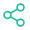Contact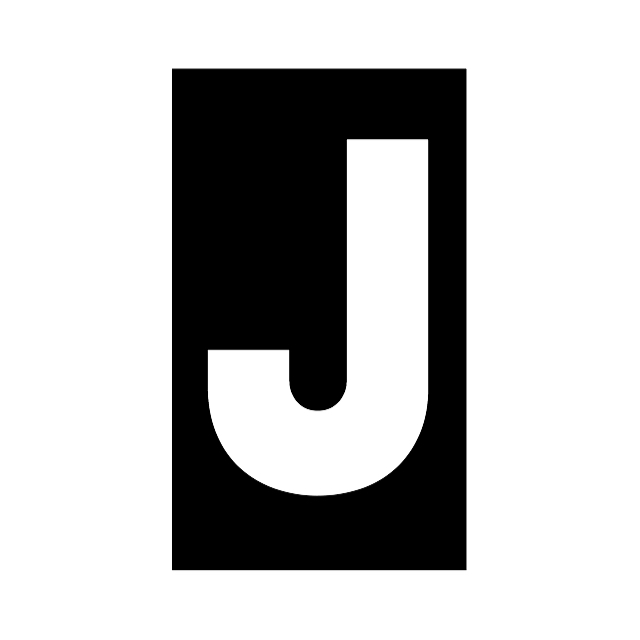0

Point(s)

(0)

Review(s)
ID : VerifyInformation propertyForeigner QuotaUseful Space :  28 Sq.m.1 Bedrooms1 BathroomsLevel 1

LocationBang Sue, Wong Sawang, Pracha ChuenCondo Regent Home Bangson Phase 2

Nearby location

•MRT Bang Son

0.1 Km.

•Big C Supercenter Wong Sawang

0.9 Km.

•Bangpo General Hospital

1.7 Km.

•Synphaet Hospital Ramintra

1.8 Km.

•King Mongkut's University of Technology North Bangkok

1.9 Km.

•Don Mueang International Airport

13.4 Km.

#### Related Post

15 minutes
3.5k
Foreigner QuotaBang Sue, Wong Sawang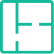28 Sq.m.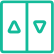Level 10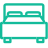1 Beds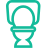1 Bath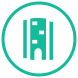Sale Down
฿2,270,00052 minutes
3k
Foreigner QuotaBang Sue, Wong Sawang56 Sq.m.Level 172 Beds2 BathFor Sale
฿1,550,0003 hours
159
Foreigner QuotaBang Sue, Wong Sawang28 Sq.m.Level 271 Beds1 Bath
3 hours
4.1k
Foreigner QuotaBang Sue, Wong Sawang56 Sq.m.Level 281 Beds2 Bath
3 hours
6.2k
Foreigner QuotaBang Sue, Wong Sawang28 Sq.m.Level 251 Beds1 Bath
3 hours
1.8k
Foreigner QuotaBang Sue, Wong Sawang61 Sq.m.Level 171 Beds2 Bath
3 hours
1.1k
Foreigner QuotaBang Sue, Wong Sawang28 Sq.m.Level 241 Beds1 BathSale Down
฿1,109,9003 hours
1.3k
Foreigner QuotaBang Sue, Wong Sawang28 Sq.m.Level 41 Beds1 Bath

# MoreLivingStock Application